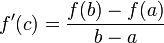# Difference between revisions of "Lagrange mean value theorem"

## Statement

Suppose$f$ is a function defined on a closed interval$[a,b]$ (with$a < b$) such that the following two conditions hold:

1.$f$ is a continuous function on the closed interval$[a,b]$ (i.e., it is right continuous at$a$, left continuous at$b$, and two-sided continuous at all points in the open interval$(a,b)$).
2.$f$ is a differentiable function on the open interval$(a,b)$, i.e., the derivative exists at all points in$(a,b)$. Note that we do not require the derivative of$f$ to be a continuous function.

Then, there exists$c$ in the open interval$(a,b)$ such that the derivative of$f$ at$c$ equals the difference quotient$\Delta f(a,b)$. More explicitly:$f'(c) = \frac{f(b) - f(a)}{b - a}$

Geometrically, this is equivalent to stating that the tangent line to the graph of$f$ at$c$ is parallel to the chord joining the points$(a,f(a))$ and$(b,f(b))$.

Note that the theorem simply guarantees the existence of$c$, and does not give a formula for finding such a$c$ (which may or may not be unique).